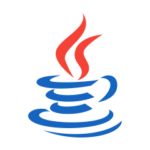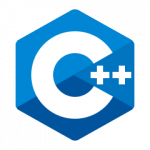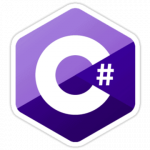## Be Calm and Keep Coding!

Start Coding today with simple2code.com and improve your coding skill. for beginners, students, and for all.
Learn Tutorials on Various Programming Languages such as C, Java, C++, and many more.

## Select the Topic## What You Can Learn ?

### Find the output ab, cd, ef, g for the input a,b,c,d,e,f,g in Javascript and Python

In this tutorial, we will write a program to find a pairs of elements from an array such that for the input [a,b,c,d,e,f,g] we will ...

### String Pattern Programs in C

In this tutorial, we will write various C pattern programs for String. Before that, you may go through the following topics in C. for loop ...

### Java Program to Find pair of Integers in Array whose sum is given Number

In this tutorial, we will write a program to find a pair of elements from an array whose sum equals a given number in java ...

### Program to Print Diamond Alphabet Patterns in C

In this tutorial, we will learn to write a C program to print Diamond patterns using alphabets/characters. However, in this tutorial, we will create a ...

### Half Diamond Pattern in C using Alphabets

In this tutorial, we will learn and code the half diamond alphabet patterns in C programming language. However, in this tutorial, we will create a ...

### Half Pyramid of Alphabets in C

In this tutorial, we will learn and code alphabet patterns in C programming language specifically the Half pyramid of alphabets in C programming. However, in ...